# 5.3 预处理数据

sklearn.preprocessing 包提供了几个常见的实用功能和变换器类型，用来将原始特征向量更改为更适合机器学习模型的形式。

## 5.3.1 标准化，也称去均值和方差按比例缩放

>>> X_train = np.array([[ 1., -1.,  2.],
...                     [ 2.,  0.,  0.],
...                     [ 0.,  1., -1.]])
...
>>> min_max_scaler = preprocessing.MinMaxScaler()
>>> X_train_minmax = min_max_scaler.fit_transform(X_train)
>>> X_train_minmax
array([[0.5       , 0.        , 1.        ],
[1.        , 0.5       , 0.33333333],
[0.        , 1.        , 0.        ]])


>>> X_scaled.mean(axis=0)
array([ 0.,  0.,  0.])

>>> X_scaled.std(axis=0)
array([ 1.,  1.,  1.])



预处理 模块还提供了一个实用类 StandardScaler ，它实现了转化器的API来计算训练集上的平均值和标准偏差，以便以后能够在测试集上重新应用相同的变换。因此，这个类适用于 sklearn.pipeline.Pipeline 的早期步骤:

>>> scaler = preprocessing.StandardScaler().fit(X_train)
>>> scaler
StandardScaler(copy=True, with_mean=True, with_std=True)

>>> scaler.mean_
array([ 1. ...,  0. ...,  0.33...])

>>> scaler.scale_
array([ 0.81...,  0.81...,  1.24...])

>>> scaler.transform(X_train)
array([[ 0.  ..., -1.22...,  1.33...],
[ 1.22...,  0.  ..., -0.26...],
[-1.22...,  1.22..., -1.06...]])



>>> X_test = [[-1., 1., 0.]]
>>> scaler.transform(X_test)
array([[-2.44...,  1.22..., -0.26...]])



### 5.3.1.1 将特征缩放至特定范围内

>>> X_train = np.array([[ 1., -1.,  2.],
...                     [ 2.,  0.,  0.],
...                     [ 0.,  1., -1.]])
...
>>> min_max_scaler = preprocessing.MinMaxScaler()
>>> X_train_minmax = min_max_scaler.fit_transform(X_train)
>>> X_train_minmax
array([[ 0.5       ,  0.        ,  1.        ],
[ 1.        ,  0.5       ,  0.33333333],
[ 0.        ,  1.        ,  0.        ]])



>>> X_test = np.array([[ -3., -1.,  4.]])
>>> X_test_minmax = min_max_scaler.transform(X_test)
>>> X_test_minmax
array([[-1.5       ,  0.        ,  1.66666667]])



>>> min_max_scaler.scale_
array([ 0.5       ,  0.5       ,  0.33...])

>>> min_max_scaler.min_
array([ 0.        ,  0.5       ,  0.33...])



X_std = (X - X.min(axis=0)) / (X.max(axis=0) - X.min(axis=0))

X_scaled = X_std * (max - min) + min



MaxAbsScaler 的工作原理非常相似，但是它只通过除以每个特征的最大值将训练数据特征缩放至 [-1, 1] 范围内，这就意味着，训练数据应该是已经零中心化或者是稀疏数据。 例子::用先前例子的数据实现最大绝对值缩放操作。

>>> X_train = np.array([[ 1., -1.,  2.],
...                     [ 2.,  0.,  0.],
...                     [ 0.,  1., -1.]])
...
>>> max_abs_scaler = preprocessing.MaxAbsScaler()
>>> X_train_maxabs = max_abs_scaler.fit_transform(X_train)
>>> X_train_maxabs                # doctest +NORMALIZE_WHITESPACE^
array([[ 0.5, -1. ,  1. ],
[ 1. ,  0. ,  0. ],
[ 0. ,  1. , -0.5]])
>>> X_test = np.array([[ -3., -1.,  4.]])
>>> X_test_maxabs = max_abs_scaler.transform(X_test)
>>> X_test_maxabs
array([[-1.5, -1. ,  2. ]])
>>> max_abs_scaler.scale_
array([ 2.,  1.,  2.])



scale 模块中进一步提供了方便的功能。当你不想创建对象时，可以使用如 minmax_scale 以及 maxabs_scale

### 5.3.1.2 缩放稀疏（矩阵）数据

MaxAbsScaler 以及 maxabs_scale 是专为缩放数据而设计的，并且是缩放数据的推荐方法。但是， scaleStandardScaler 也能够接受 scipy.sparse 作为输入，只要参数 with_mean=False 被准确传入它的构造器。否则会出现 ValueError 的错误，因为默认的中心化会破坏稀疏性，并且经常会因为分配过多的内存而使执行崩溃。 RobustScaler 不能适应稀疏输入，但你可以在稀疏输入使用 transform 方法。

### 5.3.1.3 缩放有离群值的数据

Scaling vs Whitening

## 5.3.2 非线性转换

### 5.3.2.1 映射到均匀分布

QuantileTransformer 类以及 quantile_transform 函数提供了一个基于分位数函数的无参数转换，将数据映射到了零到一的均匀分布上:

>>> from sklearn.datasets import load_iris
>>> from sklearn.model_selection import train_test_split
>>> X, y = iris.data, iris.target
>>> X_train, X_test, y_train, y_test = train_test_split(X, y, random_state=0)
>>> quantile_transformer = preprocessing.QuantileTransformer(random_state=0)
>>> X_train_trans = quantile_transformer.fit_transform(X_train)
>>> X_test_trans = quantile_transformer.transform(X_test)
>>> np.percentile(X_train[:, 0], [0, 25, 50, 75, 100])
array([ 4.3,  5.1,  5.8,  6.5,  7.9])



>>> np.percentile(X_train_trans[:, 0], [0, 25, 50, 75, 100])
...
array([ 0.00... ,  0.24...,  0.49...,  0.73...,  0.99... ])



>>> np.percentile(X_test[:, 0], [0, 25, 50, 75, 100])
...
array([ 4.4  ,  5.125,  5.75 ,  6.175,  7.3  ])
>>> np.percentile(X_test_trans[:, 0], [0, 25, 50, 75, 100])
...
array([ 0.01...,  0.25...,  0.46...,  0.60... ,  0.94...])



### 5.3.2.2 映射到高斯分布

PowerTransformer 目前提供两个这样的幂变换,Yeo-Johnson transformthe Box-Cox transform

Yeo-Johnson transform: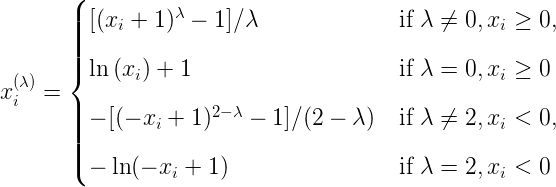Box-Cox transform: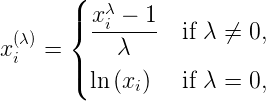Box-Cox只能应用于严格的正数据。在这两种方法中，变换都是参数化的，通过极大似然估计来确定。下面是一个使用Box-Cox将样本从对数正态分布映射到正态分布的例子:

>>> pt = preprocessing.PowerTransformer(method='box-cox', standardize=False)
>>> X_lognormal = np.random.RandomState(616).lognormal(size=(3, 3))
>>> X_lognormal
array([[1.28..., 1.18..., 0.84...],
[0.94..., 1.60..., 0.38...],
[1.35..., 0.21..., 1.09...]])
>>> pt.fit_transform(X_lognormal)
array([[ 0.49...,  0.17..., -0.15...],
[-0.05...,  0.58..., -0.57...],
[ 0.69..., -0.84...,  0.10...]])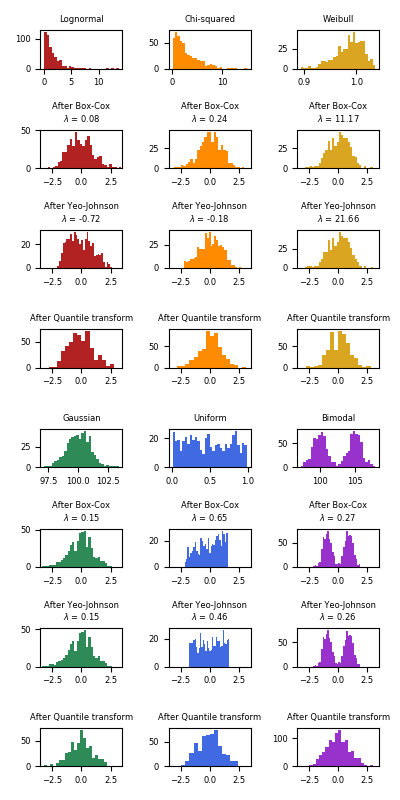>>> quantile_transformer = preprocessing.QuantileTransformer(
...     output_distribution='normal', random_state=0)
>>> X_trans = quantile_transformer.fit_transform(X)
>>> quantile_transformer.quantiles_
array([[4.3, 2. , 1. , 0.1],
[4.4, 2.2, 1.1, 0.1],
[4.4, 2.2, 1.2, 0.1],
...,
[7.7, 4.1, 6.7, 2.5],
[7.7, 4.2, 6.7, 2.5],
[7.9, 4.4, 6.9, 2.5]])


## 5.3.3 归一化

>>> X = [[ 1., -1.,  2.],
...      [ 2.,  0.,  0.],
...      [ 0.,  1., -1.]]
>>> X_normalized = preprocessing.normalize(X, norm='l2')

>>> X_normalized
array([[ 0.40..., -0.40...,  0.81...],
[ 1.  ...,  0.  ...,  0.  ...],
[ 0.  ...,  0.70..., -0.70...]])



preprocessing 预处理模块提供的 Normalizer 工具类使用 Transformer API 实现了相同的操作(即使在这种情况下， fit 方法是无用的：该类是无状态的，因为该操作独立对待样本).

>>> normalizer = preprocessing.Normalizer().fit(X)  # fit does nothing
>>> normalizer
Normalizer(copy=True, norm='l2')



>>> normalizer.transform(X)
array([[ 0.40..., -0.40...,  0.81...],
[ 1.  ...,  0.  ...,  0.  ...],
[ 0.  ...,  0.70..., -0.70...]])

>>> normalizer.transform([[-1.,  1., 0.]])
array([[-0.70...,  0.70...,  0.  ...]])



## 5.3.4 类别特征编码

>>> enc = preprocessing.OrdinalEncoder()
>>> X = [['male', 'from US', 'uses Safari'], ['female', 'from Europe', 'uses Firefox']]
>>> enc.fit(X)
OrdinalEncoder(categories='auto', dtype=<... 'numpy.float64'>)
>>> enc.transform([['female', 'from US', 'uses Safari']])
array([[0., 1., 1.]])


>>>
>>> enc = preprocessing.OneHotEncoder()
>>> X = [['male', 'from US', 'uses Safari'], ['female', 'from Europe', 'uses Firefox']]
>>> enc.fit(X)
OneHotEncoder(categorical_features=None, categories=None,
dtype=<... 'numpy.float64'>, handle_unknown='error',
n_values=None, sparse=True)
>>> enc.transform([['female', 'from US', 'uses Safari'],
...                ['male', 'from Europe', 'uses Safari']]).toarray()
array([[1., 0., 0., 1., 0., 1.],
[0., 1., 1., 0., 0., 1.]])


>>>
>>> enc.categories_
[array(['female', 'male'], dtype=object), array(['from Europe', 'from US'], dtype=object), array(['uses Firefox', 'uses Safari'], dtype=object)]


>>> genders = ['female', 'male']
>>> locations = ['from Africa', 'from Asia', 'from Europe', 'from US']
>>> browsers = ['uses Chrome', 'uses Firefox', 'uses IE', 'uses Safari']
>>> enc = preprocessing.OneHotEncoder(categories=[genders, locations, browsers])
>>> # Note that for there are missing categorical values for the 2nd and 3rd
>>> # feature
>>> X = [['male', 'from US', 'uses Safari'], ['female', 'from Europe', 'uses Firefox']]
>>> enc.fit(X)
OneHotEncoder(categorical_features=None,
categories=[...], drop=None,
dtype=<... 'numpy.float64'>, handle_unknown='error',
n_values=None, sparse=True)
>>> enc.transform([['female', 'from Asia', 'uses Chrome']]).toarray()
array([[1., 0., 0., 1., 0., 0., 1., 0., 0., 0.]])


>>> enc = preprocessing.OneHotEncoder(handle_unknown='ignore')
>>> X = [['male', 'from US', 'uses Safari'], ['female', 'from Europe', 'uses Firefox']]
>>> enc.fit(X)
OneHotEncoder(categorical_features=None, categories=None, drop=None,
dtype=<... 'numpy.float64'>, handle_unknown='ignore',
n_values=None, sparse=True)
>>> enc.transform([['female', 'from Asia', 'uses Chrome']]).toarray()
array([[1., 0., 0., 0., 0., 0.]])


>>> X = [['male', 'from US', 'uses Safari'], ['female', 'from Europe', 'uses Firefox']]
>>> drop_enc = preprocessing.OneHotEncoder(drop='first').fit(X)
>>> drop_enc.categories_
[array(['female', 'male'], dtype=object), array(['from Europe', 'from US'], dtype=object), array(['uses Firefox', 'uses Safari'], dtype=object)]
>>> drop_enc.transform(X).toarray()
array([[1., 1., 1.],
[0., 0., 0.]])


## 5.3.5 离散化

One-hot 编码的离散化特征 可以使得一个模型更加的有表现力(expressive)，同时还能保留其可解释性(interpretability)。 比如，用离散化器进行预处理可以给线性模型引入非线性。

### 5.3.5.1 K-bins 离散化

KBinsDiscretizer 类使用k个等宽的bins把特征离散化

>>> X = np.array([[ -3., 5., 15 ],
...               [  0., 6., 14 ],
...               [  6., 3., 11 ]])
>>> est = preprocessing.KBinsDiscretizer(n_bins=[3, 2, 2], encode='ordinal').fit(X)


>>> est.transform(X)
array([[ 0., 1., 1.],
[ 1., 1., 1.],
[ 2., 0., 0.]])


KBinsDiscretizer类实现了不同的 binning策略，可以通过参数strategy进行选择。 ‘uniform’ 策略使用固定宽度的bins。 ‘quantile’ 策略在每个特征上使用分位数(quantiles)值以便具有相同填充的bins。 ‘kmeans’ 策略基于在每个特征上独立执行的k-means聚类过程定义bins。

### 5.3.5.2 特征二值化

>>> X = [[ 1., -1.,  2.],
...      [ 2.,  0.,  0.],
...      [ 0.,  1., -1.]]

>>> binarizer = preprocessing.Binarizer().fit(X)  # fit does nothing
>>> binarizer
Binarizer(copy=True, threshold=0.0)

>>> binarizer.transform(X)
array([[ 1.,  0.,  1.],
[ 1.,  0.,  0.],
[ 0.,  1.,  0.]])



>>> binarizer = preprocessing.Binarizer(threshold=1.1)
>>> binarizer.transform(X)
array([[ 0.,  0.,  1.],
[ 1.,  0.,  0.],
[ 0.,  0.,  0.]])



binarize 以及 Binarizer 接收 来自scipy.sparse的密集类数组数据以及稀疏矩阵作为输入

## 5.3.7 生成多项式特征

>>> import numpy as np
>>> from sklearn.preprocessing import PolynomialFeatures
>>> X = np.arange(6).reshape(3, 2)
>>> X
array([[0, 1],
[2, 3],
[4, 5]])
>>> poly = PolynomialFeatures(2)
>>> poly.fit_transform(X)
array([[  1.,   0.,   1.,   0.,   0.,   1.],
[  1.,   2.,   3.,   4.,   6.,   9.],
[  1.,   4.,   5.,  16.,  20.,  25.]])



X 的特征已经从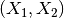转换为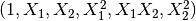>>> X = np.arange(9).reshape(3, 3)
>>> X
array([[0, 1, 2],
[3, 4, 5],
[6, 7, 8]])
>>> poly = PolynomialFeatures(degree=3, interaction_only=True)
>>> poly.fit_transform(X)
array([[   1.,    0.,    1.,    2.,    0.,    0.,    2.,    0.],
[   1.,    3.,    4.,    5.,   12.,   15.,   20.,   60.],
[   1.,    6.,    7.,    8.,   42.,   48.,   56.,  336.]])



X的特征已经从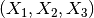转换为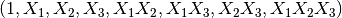## 5.3.8 自定义转换器

>>> import numpy as np
>>> from sklearn.preprocessing import FunctionTransformer
>>> transformer = FunctionTransformer(np.log1p, validate=True)
>>> X = np.array([[0, 1], [2, 3]])
>>> transformer.transform(X)
array([[0.        , 0.69314718],
[1.09861229, 1.38629436]])